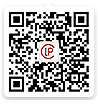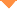首页 > 论文 > 光学学报 > 40卷 > 20期(pp：2026001--1)

Detection of Rotational Cylinder Speed Using Doppler Effect of Optical Vortex

• 摘要• 论文信息• 参考文献• 被引情况• PDF全文Abstract

As a structured beam exhibiting a spiral phase and an orbital angular momentum, the Doppler effect of an optical vortex possesses a frequency shift component caused by the relative motion that is perpendicular to the beam cross-section compared with the Doppler effect of the classical plane beam. In this study, a velocity-Poynting vector model and a phase modulation model are established to study the linear and rotational Doppler effects of optical vortex, obtaining the generation law of Doppler frequency shift of the vortex light under compound motion. Moreover, these two models are used to analyze the Doppler frequency shift on the surface of the cylinder, which is a typical spatial moving target. The same results are obtained, proving the correctness of the proposed theoretical analysis method. The distribution law of the frequency shift of the rotating cylinder detected through vortex light is proposed herein, and the influence of different optical vortex radii and cylinder sizes on the detection results is explored.【1】Censor D. Theory of the Doppler effect: fact, fiction and approximation [J]. Radio Science. 1984, 19(4): 1027-1040.Censor D. Theory of the Doppler effect: fact, fiction and approximation [J]. Radio Science. 1984, 19(4): 1027-1040.

【3】Varghese B. Rajan V, van LeeuwenT G, et al. Discrimination between Doppler-shifted and non-shifted light in coherence domain path length resolved measurements of multiply scattered light [J]. Optics Express. 2007, 15(20): 13340-13350.

【4】Coullet P, Gil L, Rocca F. Optical vortices [J]. Optics Communications. 1989, 73(5): 403-408.

【5】Allen L, Beijersbergen M W. Spreeuw R J C, et al. Orbital angular momentum of light and the transformation of Laguerre-Gaussian laser modes [J]. Physical Review A. 1992, 45(11): 8185-8189.

【6】Wang C, Liu T, Ren Y, et al. Generating optical vortex with large topological charges by spiral phase plates in cascaded and double-pass configuration [J]. Optik. 2018, 171: 404-412.

【7】Padgett M J. Orbital angular momentum 25 years on [Invited] [J]. Optics Express. 2017, 25(10): 11265-11274.

【9】Poynting J H. The wave motion of a revolving shaft, and a suggestion as to the angular momentum in a beam of circularly polarised light [J]. Proceedings of the Royal Society A. 1909, 82(557): 560-567.

【10】Padgett M J, Allen L. The Poynting vector in Laguerre-Gaussian laser modes [J]. Optics Communications. 1995, 121(1/2/3): 36-40.

【11】Leach J, Keen S, Padgett M J, et al. Direct measurement of the skew angle of the Poynting vector in a helically phased beam [J]. Optics Express. 2006, 14(25): 11919-11924.

【12】Allen L, Babiker M, Power W L. Azimuthal Doppler shift in light beams with orbital angular momentum [J]. Optics Communications. 1994, 112(3/4): 141-144.

【13】Garetz B A. Angular Doppler effect [J]. Journal of the Optical Society of America. 1981, 71(5): 609-611.

【14】Qi Q Q, Chen C B, Xu T Z, et al. Optimal measurement of rotation Doppler-frequency shifts based on rotational symmetry [J]. Journal of Xiamen University (Natural Science). 2017, 56(2): 220-225.

【15】Fu S Y, Wang T L, Zhang Z Y, et al. Non-diffractive Bessel-Gauss beams for the detection of rotating object free of obstructions [J]. Optics Express. 2017, 25(17): 20098-20108.

【17】Hu X B, Zhao B, Zhu Z H, et al. In situ detection of a cooperative target''''s longitudinal and angular speed using structured light [J]. Optics Letters. 2019, 44(12): 3070-3073.

【18】Lavery M P J, Speirits F C, Barnett S M, et al. Detection of a spinning object using light''''s orbital angular momentum [J]. Science. 2013, 341(6145): 537-540.

【19】Lavery M P J, Barnett S M, Speirits F C, et al. Observation of the rotational Doppler shift of a white-light, orbital-angular-momentum-carrying beam backscattered from a rotating body [J]. Optica. 2014, 1(1): 1-4.

【20】Fang L, Padgett M J, Wang J. Sharing a common origin between the rotational and linear Doppler effects [J]. Laser & Photonics Reviews. 2017, 11(6): 1770064.

【21】Liu B Y, Chu H C, Giddens H, et al. Experimental observation of linear and rotational Doppler shifts from several designer surfaces [J]. Scientific Reports. 2019, 9(1): 8971.

【22】Qiu S, Liu T, Li Z M, et al. Influence of lateral misalignment on the optical rotational Doppler effect [J]. Applied Optics. 2019, 58(10): 2650-2655.

【23】Qiu S, Liu T, Ren Y, et al. Detection of spinning objects at oblique light incidence using the optical rotational Doppler effect [J]. Optics Express. 2019, 27(17): 24781-24792.

【24】Belmonte A, Torres J P. Optical Doppler shift with structured light [J]. Optics Letters. 2011, 36(22): 4437-4439.

【25】Anderson A Q, Strong E F, Heffernan B M, et al. Detection technique effect on rotational Doppler measurements [J]. Optics Letters. 2020, 45(9): 2636-2639.

Qiu Song,Ren Yuan,Liu Tong,Wang Chen. Detection of Rotational Cylinder Speed Using Doppler Effect of Optical Vortex[J]. Acta Optica Sinica, 2020, 40(20): 2026001